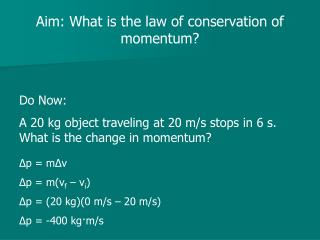DownloadDownload PresentationAim: What is the law of conservation of momentum?

# Aim: What is the law of conservation of momentum?

Download Presentation## Aim: What is the law of conservation of momentum?

- - - - - - - - - - - - - - - - - - - - - - - - - - - E N D - - - - - - - - - - - - - - - - - - - - - - - - - - -
##### Presentation Transcript

1. Aim: What is the law of conservation of momentum? Do Now: A 20 kg object traveling at 20 m/s stops in 6 s. What is the change in momentum? Δp = mΔv Δp = m(vf – vi) Δp = (20 kg)(0 m/s – 20 m/s) Δp = -400 kg·m/s

2. Newton’s cradle demo How can we explain what is going on? The momentum of: one goes in, one goes out two goes in, two goes out etc.

3. The Law of Conservation of Momentum The momentum of any closed, isolated system does not change.

4. A 2,000 kg car traveling north at 3 m/s strikes a 3,000 kg car traveling south at 2 m/s. What is the final momentum after the cars collide? pi = pf p1i + p2i = pf mv1i + mv2i = pf (2,000 kg)(3 m/s) + (3,000 kg)(-2 m/s) = pf 6,000 kg·m/s – 6,000 kg·m/s = pf pf = 0 kg·m/s

5. Spring or Gun When firing a gun, there will always be a recoil due to momentum being conserved http://www.youtube.com/watch?v=sTHPfz0bVycA 5 kg gun fires a 0.02 kg bullet. If the bullet exits the gun at 800 m/s East, calculate the recoil velocity of the gun. Rifle Recoil Video

6. Inelastic Collision A collision where the objects stick together after the collision • Masses combine • Velocity decreases after the collision

7. A 1 kg cart traveling at 1 m/s to the right strikes a 0.7 kg cart initially at rest. After the collision, the two stick together. Calculate the final velocity of the two cart system. pi = pf p1i + p2i = p(1+2)f mv1i + mv2i = (m1 + m2)vf (1 kg)(1 m/s) + (0.7 kg)(0 m/s) = (1 kg + 0.7 kg)vf 1 + 0 = 1.7vf 1 = 1.7vf vf = 0.59 m/s right

8. Elastic Collision Collision where the objects bounce off each other

9. Mass 1 (1 kg) is traveling at 1 m/s to the right and strikes mass 2 (1 kg) that is at rest. After the collision, mass 1 is at rest. What is the velocity of the mass 2? pi = pf p1i + p2i = p1f + p2f m1v1i + m2v2i = m1v1f + m2v2f (1 kg)(1 m/s) + (1 kg)(0 m/s) = (1 kg)(0 m/s) +(1 kg)v2f 1 + 0 = 0 + 1v2f v2f = 1 m/s right

10. What if a heavier objects strikes a lighter object? • Both will continue to move in the same direction • The heavier object will slow down

11. Mass 1 (1 kg) is traveling at 1 m/s to the right and strikes mass 2 (0.7 kg) that is at rest. After the collision, mass 1 is traveling at 0.18 m/s to the right. What is the velocity of the mass 2? pi = pf p1i + p2i = p1f + p2f m1v1i + m2v2i = m1v1f + m2v2f (1 kg)(1 m/s) + (0.7 kg)(0 m/s) = (1 kg)(0.18 m/s) +(0.7 kg)v2f 1 + 0 = 0.18 + 0.7v2f 0.82 = 0.7v2f v2f = 1.17 m/s right

12. What if a lighter object strikes a heavier object? • The lighter object will bounce off in the opposite direction

13. Mass 1 (1 kg) is traveling at 1 m/s to the right and strikes mass 2 (1.4 kg) that is at rest. After the collision, mass 2 is traveling at 0.83 m/s to the right. What is the velocity of the mass 1? pi = pf p1i + p2i = p1f + p2f m1v1i + m2v2i = m1v1f + m2v2f (1 kg)(1 m/s) + (1.4 kg)(0 m/s) = (1 kg)v1f +(1.4 kg)(0.83 m/s) 1 + 0 = v1f + 1.2 v1f = -0.2 m/s or 0.2 m/s left